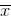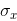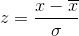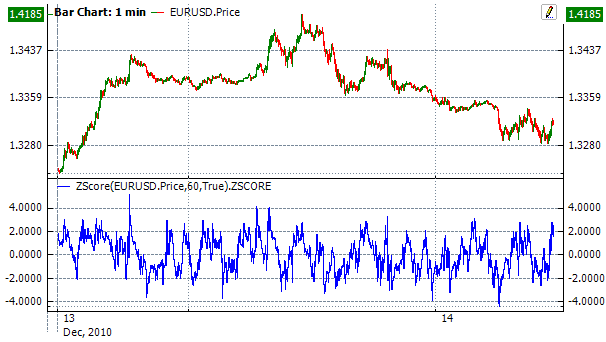﻿ZScore

# ZScore

The Z-Score (or standard score ) (ZScore) is a numerical measurement of a value's relationship to the mean (average) of a group of values, measured in terms of standard deviations from the mean. If a Z-score is 0, it indicates that the data point's score is identical to the mean score. A Z-score of 1.0 would indicate a value that is one standard deviation from the mean. Z-scores may be positive or negative, with a positive value indicating the score is above the mean and a negative score indicating it is below the mean.Calculation

It is calculated by subtracting the meanfrom an individual raw score and then dividing the difference by the standard deviation:Chart ExampleImplementation and Usage

To initialize indicator, use one of the following constructors:

ZScore – set default values: period = 14

ZScore(Int32) – set value for period

ZScore(TimeSpan) – sets time period

Use

ZSCORE - property to get current valueExample
C#
``` 1// Create new instance
2var indicator = new ZScore(28);
3
4// Number of stored values
5indicator.HistoryCapacity = 2;
6